# Langmuir probe VA characteristics measurement of the standard GOLEM tokamak plasma

Aim: to measure VA characteristics on the shot to shot basis.

##Experimental setup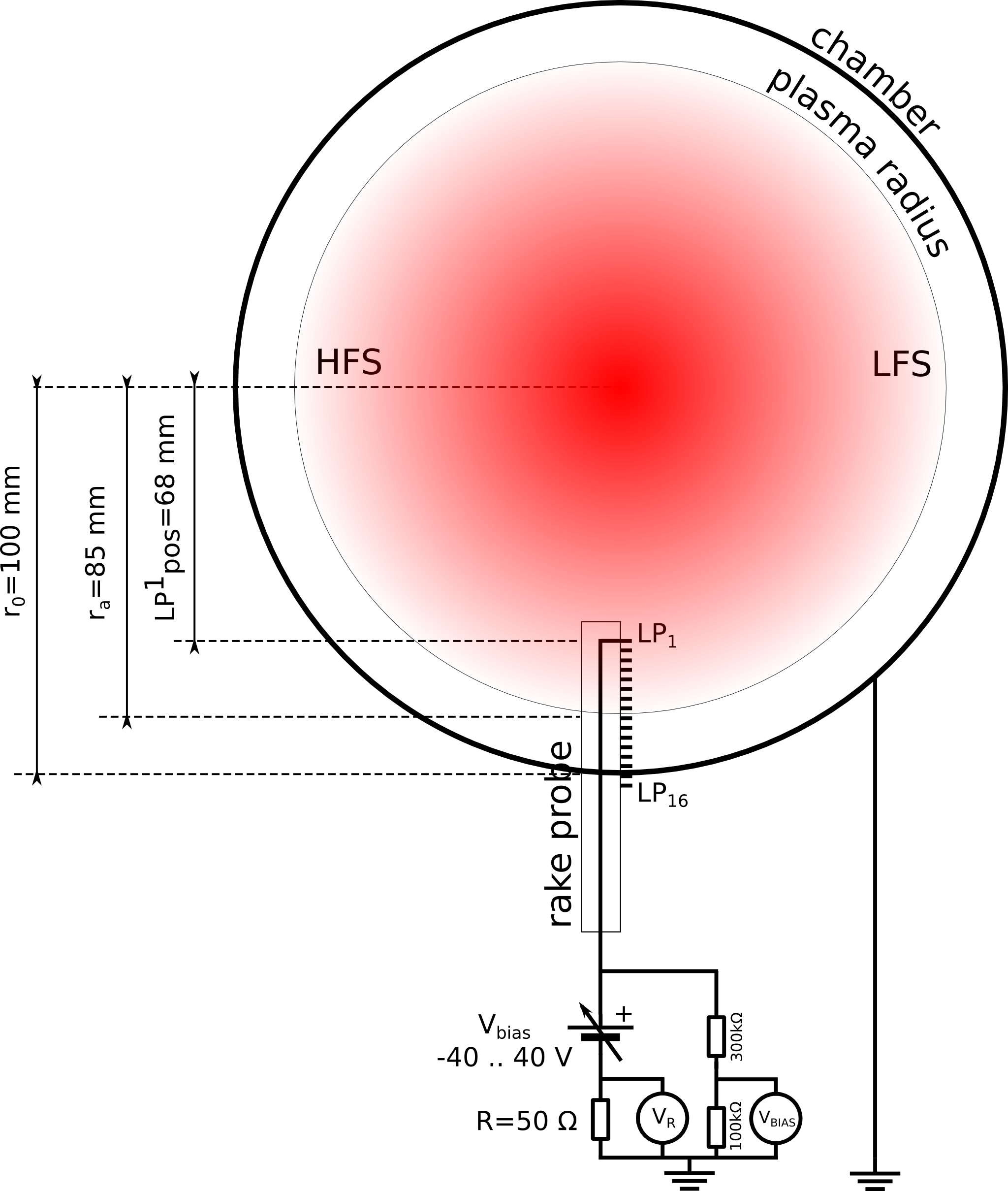Rake probe description

##Theory

###Initial configuration:

• Date: Afternoon 261114
• LP position of 1st pin from the vessel center $$LP_{pos}=107$$ mm,
• $$V_{bias}$$ off,
• $$R=100$$ Ohm:
• LP orientation: pins ahead SouthEast (in the port direction), i.e. probe is oriented perpendicularly to the magnetic field lines
• Discharge setup: –UBt 800 –Ucd 500 –Tcd 10000 –Tres 2000 –pressure 1 –preionization 4
• Preionization: ECR wave
• $$V_{bias}$$ measured with 1:100 divider

###Minutes of the experiment:

Reference shot #17717
Switching on $$V_{bias}$$ to ~ 10V #17718 OK, $$V_{bias}$$ goes to ~0.1V
Lp to position $$LP_{pos}$$ = 54 mm #17719 $$I_{sat}$$ too high ~ 100 mA
Galvanic insulation implemented for V_bias power supply #17720 Satisfactory, no problem
Since $$I_{sat}$$ is too high,
Let’s decrease R to 50 Ohm
#17721 Better, but still $$V_R$$ ~9V
Since $$I_{sat}$$ is still too high,
Let’s take out LP to position 68 mm
#17722 to #17726 fails
#17727 OK No effect, still $$V_R$$ ~9V
HS discussion: configuration OK
reverse $$V_{bias}$$ polarity to -10 V to measure $$I_{is}$$
#17728 to #17733 fails
#17734 OK Hopefully good result
$$V_{bias}$$ = {-20,-30,-40} V #17735,#17736,#17737 OK, ready for scan
Scan $$V_{bias}$$ = {-40,-35,-30,-25,-20,-15,-10,-5,0,1,2,3,4,5,6,7,8,9,10,12} V From #17739 to #17762 see Figs. 1, 2, 3, 4

###Final experiment configuration:

• LP position of 1st pin from the vessel center $$LP_{pos}=68$$ mm,
• $$V_{bias}$$ from -40V (#17739) to 12V (#17762),
• $$R=50$$ Ohm:
• LP orientation: pins ahead SouthEast (in the port direction) i.e. probe is oriented perpendicularly to the magnetic field lines
• Discharge setup: –UBt 800 –Ucd 500 –Tcd 10000 –Tres 2000 –pressure 1 –preionization 4
• Preionization: ECR wave
• $$V_{bias}$$ measured with 1:100 divider
• Length of pin: 2 mm, diameter of the pin: 0.7 mm => pin surface ~ 3.140.7210^-6 m^2 ~ 4.410^-6 m^2

##Reference shot #17743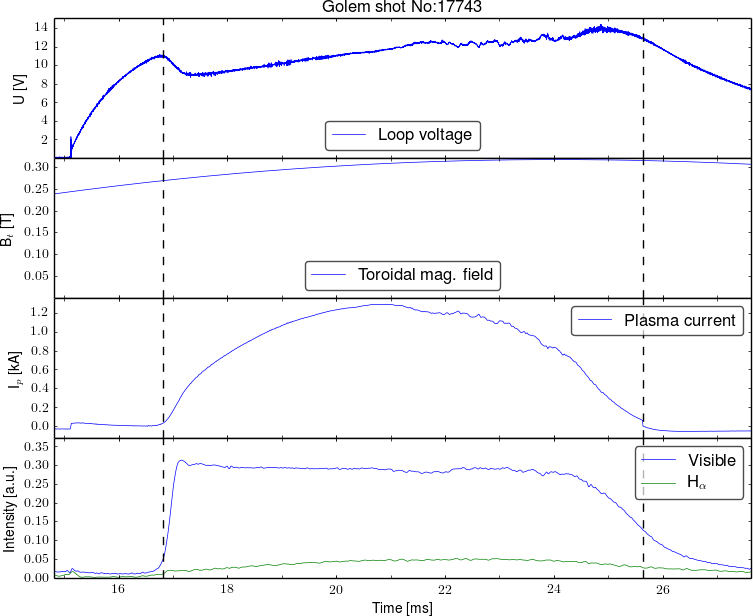Reproducibility of the GOLEM tokamak discharge from #17739 to #17762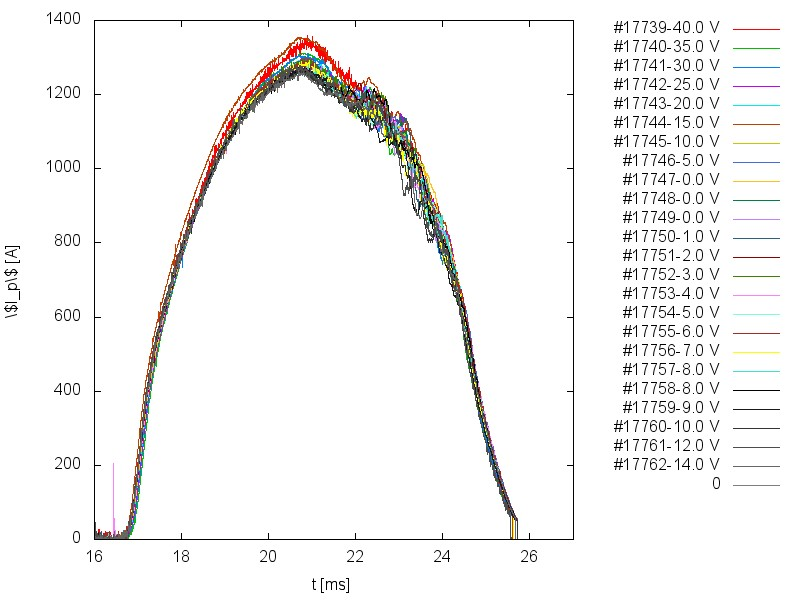$$I_{p}$$ scan - alltogether (jpg, svg,canvas,script)

##Raw experimental data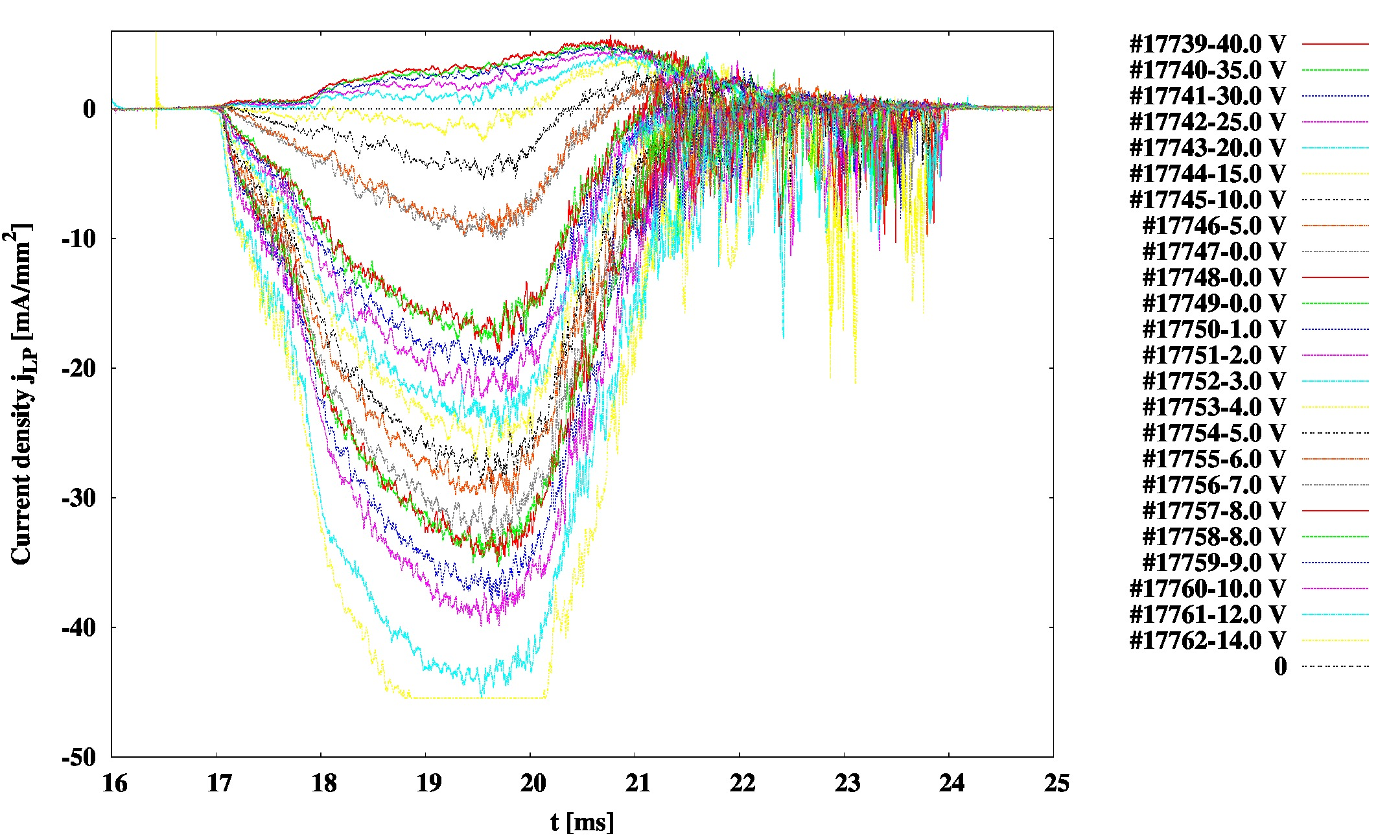Fig.1: $$V_{bias}$$ scan - alltogether (jpg, svg,canvas,script)Fig.2: $$V_{bias}$$ scan - ion side (jpg, svg,canvas,script)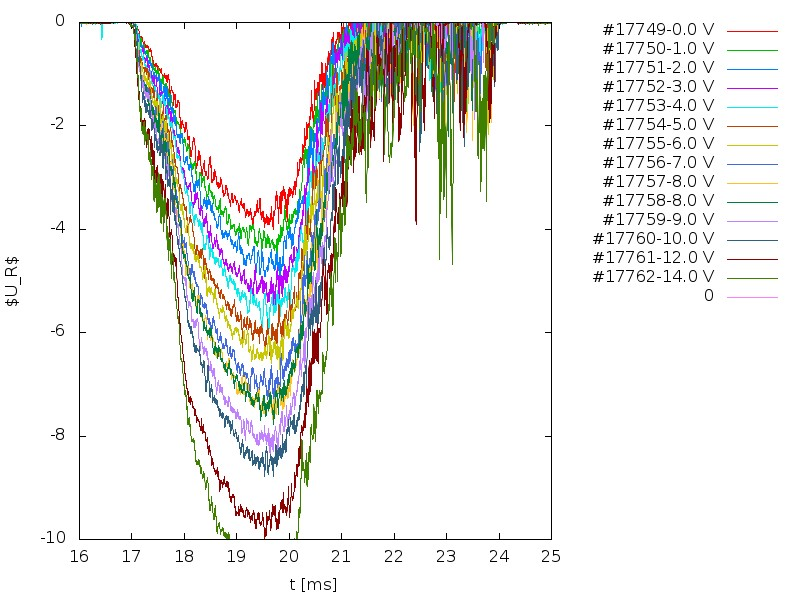Fig.3: $$V_{bias}$$ scan - electron side (jpg, svg,canvas,script)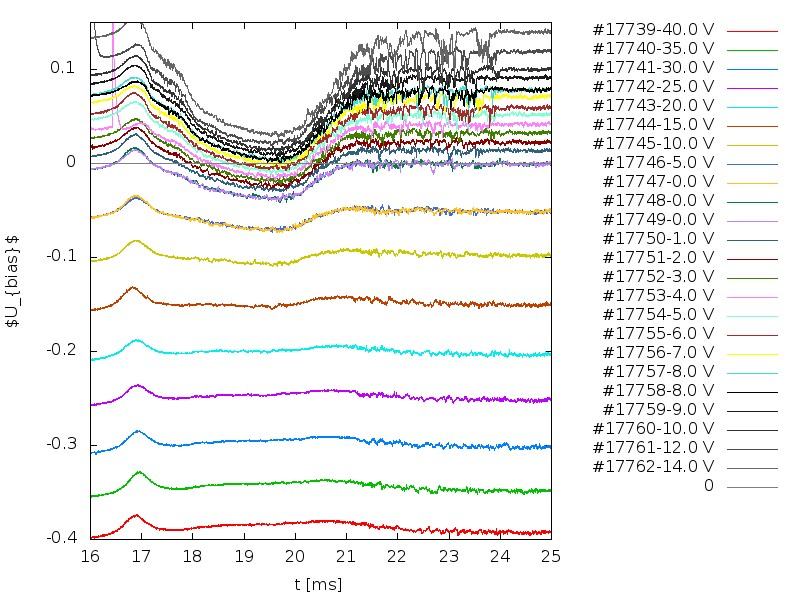Fig.4: $$V_{bias}$$ scan - alltogether $$U_{bias}$$ (jpg, svg,canvas,script)

##Analysis ### Raw data - VA characteristics at specific time with $$10\mu s$$ averaging.

### VA characteristics fit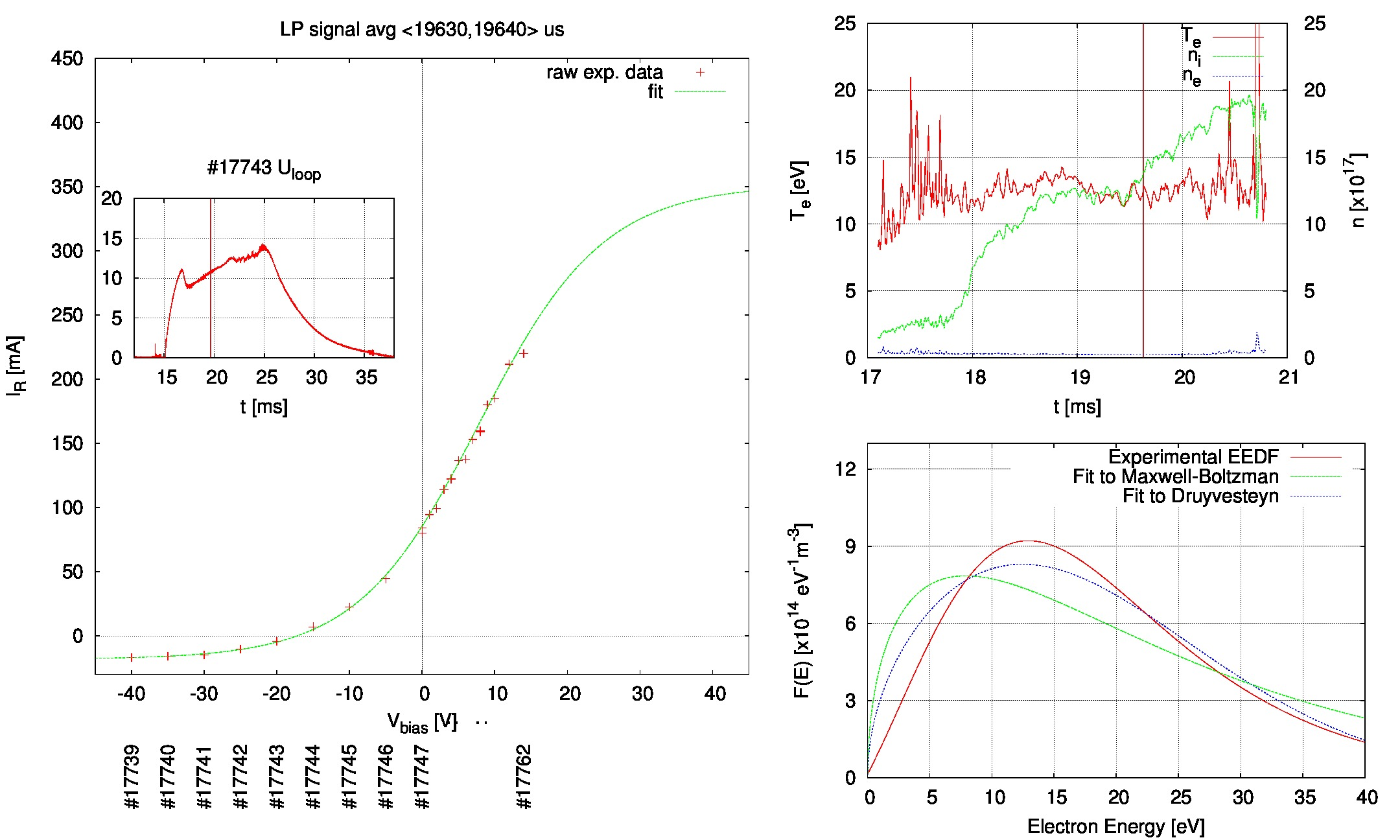This video in avi format

## Discussion

• The reason for the VA char vanish in the end of the discharge could be fact, that the GOLEM plasma is normally moving up as can be seen here (#11405):Note the rake probe seen in the photo.

## EEDF

• [Annette Meiners @COMSOL: Electron Energy Distribution Function](http://www.comsol.com/blogs/electron-energy-distribution-function/)